The POWER Procedure

### Analyses in the TWOSAMPLEWILCOXON Statement

#### Wilcoxon-Mann-Whitney Test for Comparing Two Distributions (TEST=WMW)

The power approximation in this section is applicable to the Wilcoxon-Mann-Whitney (WMW) test as invoked with the WILCOXON option in the PROC NPAR1WAY statement of the NPAR1WAY procedure. The approximation is based on O’Brien and Castelloe (2006) and an estimator called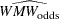. See O’Brien and Castelloe (2006) for a definition of, which need not be derived in detail here for purposes of explaining the power formula.

Let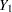and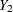be independent observations from any two distributions that you want to compare using the WMW test. For purposes of deriving the asymptotic distribution of(and consequently the power computation as well), these distributions must be formulated as ordered categorical ("ordinal") distributions.

If a distribution is continuous, it can be discretized using a large number of categories with negligible loss of accuracy. Each nonordinal distribution is divided intocategories, whereis the value of the NBINS parameter, with breakpoints evenly spaced on the probability scale. That is, each bin contains an equal probability/for that distribution. Then the breakpoints across both distributions are pooled to form a collection of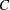bins (heretofore called "categories"), and the probabilities of bin membership for each distribution are recalculated. The motivation for this method of binning is to avoid degenerate representations of the distributions—that is, small handfuls of large probabilities among mostly empty bins—as can be caused by something like an evenly spaced grid across raw values rather than probabilities.

After the discretization process just mentioned, there are now two ordinal distributions, each with a set of probabilities across a common set ofordered categories. For simplicity of notation, assume (without loss of generality) the response values to be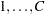. Represent the conditional probabilities as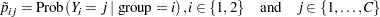and the group allocation weights as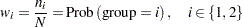The joint probabilities can then be calculated simply as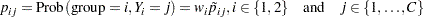The next step in the power computation is to compute the probabilities that a randomly chosen pair of observations from the two groups is concordant, discordant, or tied. It is useful to define these probabilities as functions of the terms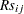and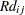, defined as follows, where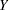is a random observation drawn from the joint distribution across groups and categories: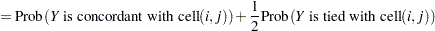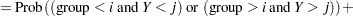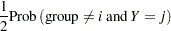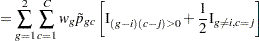and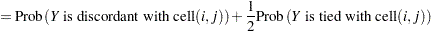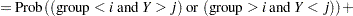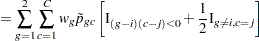For an independent random draw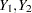from the two distributions,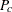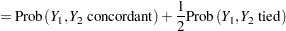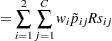and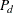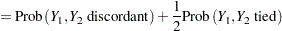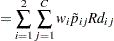Then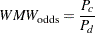Proceeding to compute the theoretical standard error associated with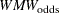(that is, the population analogue to the sample standard error),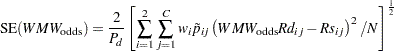Converting to the natural log scale and using the delta method,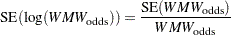The next step is to produce a "smoothed" version of the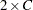cell probabilities that conforms to the null hypothesis of the Wilcoxon-Mann-Whitney test (in other words, independence in thecontingency table of probabilities). Let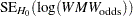denote the theoretical standard error of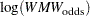assuming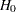.

Finally we have all of the terms needed to compute the power, using the noncentral chi-square and normal distributions: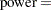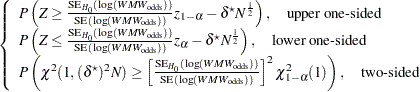where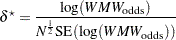is the primary noncentrality—that is, the "effect size" that quantifies how much the two conjectured distributions differ.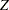is a standard normal random variable,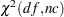is a noncentral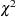random variable with degrees of freedom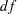and noncentrality, and
is the total sample size.Previous Page | Next Page | Top of Page